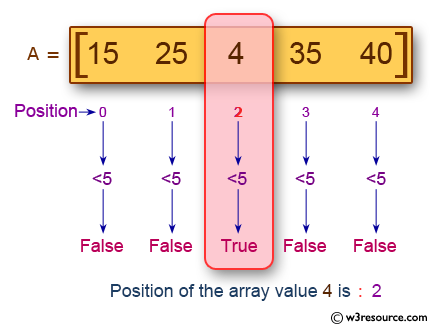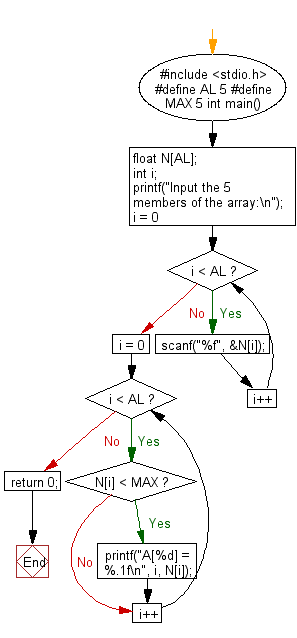﻿ C Program: Print the position and value of an array elements - w3resource

# C Exercises: Print the position and value of an array elements

## C Basic Declarations and Expressions: Exercise-50 with Solution

Write a C program to read an array of length 5 and print the position and value of the array elements of value less than 5.

Pictorial Presentation:Sample Solution:

C Code:

``````#include <stdio.h>
#define AL 5  // Define the size of the array as 5
#define MAX 5 // Define a maximum value for comparison

int main() {
float N[AL]; // Declare an array of size 5 to hold floating-point numbers
int i;

// Prompt for user input
printf("Input the 5 members of the array:\n");

// Read and store user input into the array
for(i = 0; i < AL; i++) {
scanf("%f", &N[i]);
}

// Iterate through the array and print elements less than MAX
for(i = 0; i < AL; i++) {
if(N[i] < MAX) {
printf("A[%d] = %.1f\n", i, N[i]);
}
}

return 0;
}
``````

Sample Output:

```Input the 5 members of the array:
15
25
4
35
40
A = 4.0
```

Flowchart:C programming Code Editor:

What is the difficulty level of this exercise?

Test your Programming skills with w3resource's quiz.

﻿